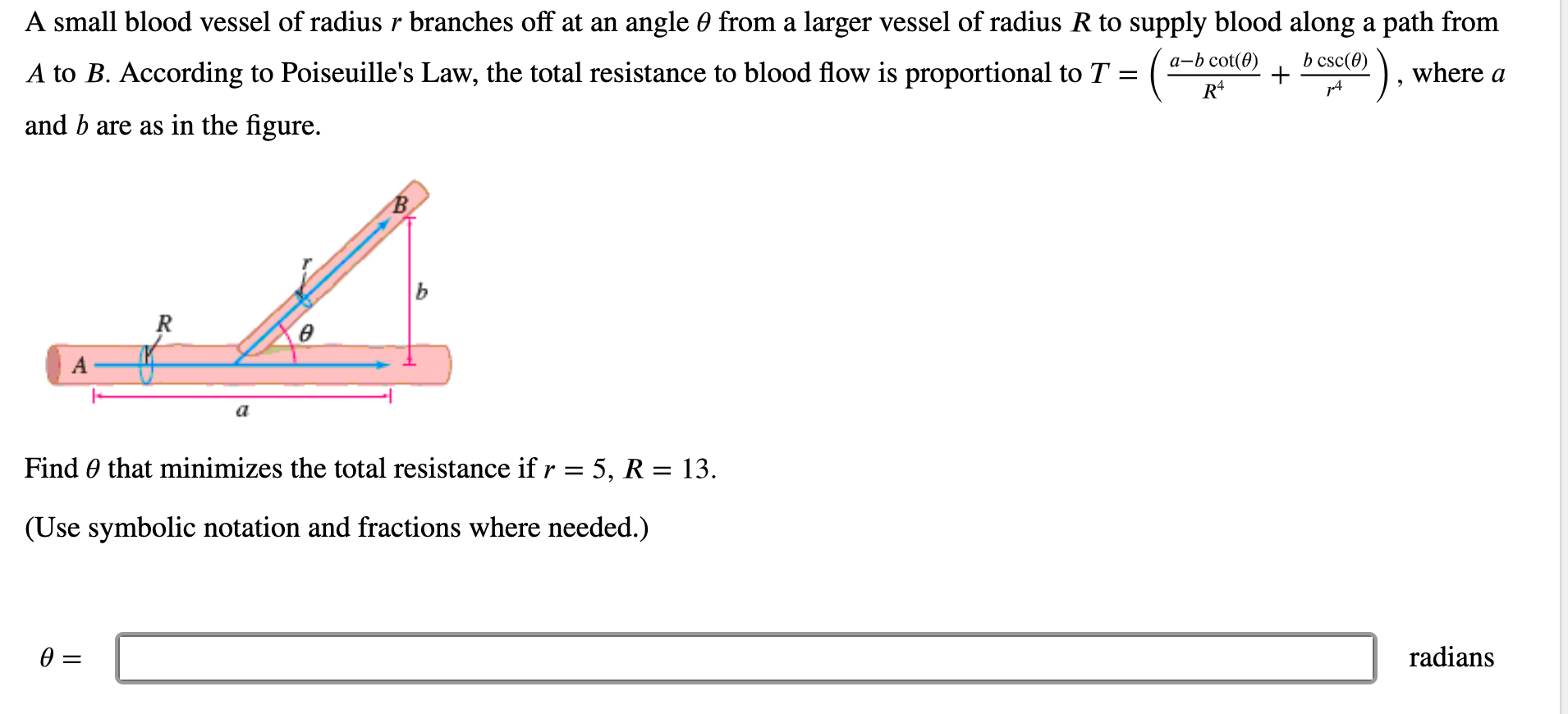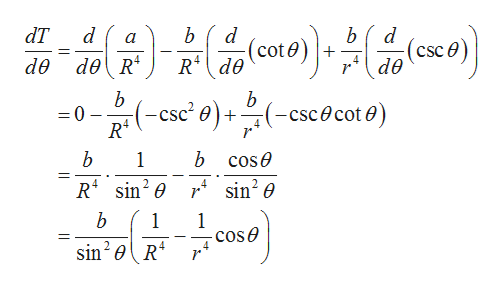# A small blood vessel of radius r branches off at an angle 0 from a larger vessel of radius R to supply blood along a path fromа-b cot(0)b csc(0)A to B. According to Poiseuille's Law, the total resistance to blood flow is proportional to T = ( -where aand b are as in the figure.В.ьRFind 0 that minimizes the total resistance if r = 5, R = 13.(Use symbolic notation and fractions where needed.)radians

Question
128 viewshelp_outlineImage TranscriptioncloseA small blood vessel of radius r branches off at an angle 0 from a larger vessel of radius R to supply blood along a path from а-b cot(0) b csc(0) A to B. According to Poiseuille's Law, the total resistance to blood flow is proportional to T = ( - where a and b are as in the figure. В. ь R Find 0 that minimizes the total resistance if r = 5, R = 13. (Use symbolic notation and fractions where needed.) radians fullscreen
check_circle

star
star
star
star
star
1 Rating
Step 1

It is given that the total blood resistance is proportional to T.

Step 2

Differentiate the above equation to min...help_outlineImage TranscriptionclosedT d ot R2de b b d csce de a de deR b 0 R2 b (-cscecote (-sc' e)scecot 0) 4 b cose b 1 sin2 e 4 b 1 1 - cos e sin20R 4 fullscreen

### Want to see the full answer?

See Solution

#### Want to see this answer and more?

Solutions are written by subject experts who are available 24/7. Questions are typically answered within 1 hour.*

See Solution
*Response times may vary by subject and question.
Tagged in

### Calculus